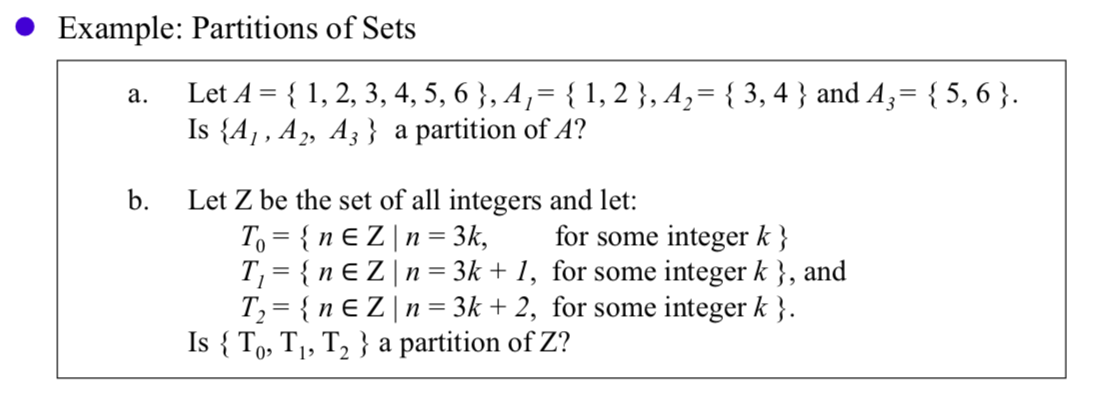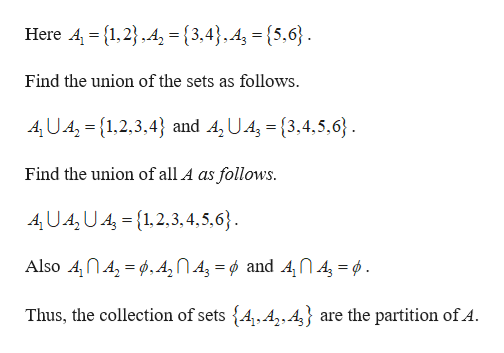# Example: Partitions of SetsLet A 1, 2, 3, 4, 5, 6}, A,= {1,2}, A,= {3,4} and A= {5, 6}Is {A1, A2, A3} a partition of A?а.b.Let Z be the set of all integers and let:To {n E Zn = 3kT, {nE Zn = 3k + 1, for some integer k}, andT2 {n E Z n = 3k + 2, for some integer k }.Is {To, T,, T2 } a partition of Z?for some integer k}0

Question
23 views

discrete mathhelp_outlineImage TranscriptioncloseExample: Partitions of Sets Let A 1, 2, 3, 4, 5, 6}, A,= {1,2}, A,= {3,4} and A= {5, 6} Is {A1, A2, A3} a partition of A? а. b. Let Z be the set of all integers and let: To {n E Zn = 3k T, {nE Zn = 3k + 1, for some integer k}, and T2 {n E Z n = 3k + 2, for some integer k }. Is {To, T,, T2 } a partition of Z? for some integer k} 0 fullscreen
check_circle

Step 1

(a)

It is known that the collection of disjoints subset of a given set or if the union of the subs...help_outlineImage TranscriptioncloseHere A 1,2A{3,4},4, = {5,6} Find the union of the sets as follows 4UA, 1,2,3,4 and A, U.A(3,4,5,6} Find the union of all A as follows. AU.A2UA1,2,3,4,5,6} A2NA = and 4 I 4 Also A,nA Thus, the collection of sets {4,A,,A are the partition of A fullscreen

### Want to see the full answer?

See Solution

#### Want to see this answer and more?

Solutions are written by subject experts who are available 24/7. Questions are typically answered within 1 hour.*

See Solution
*Response times may vary by subject and question.
Tagged in

### Math